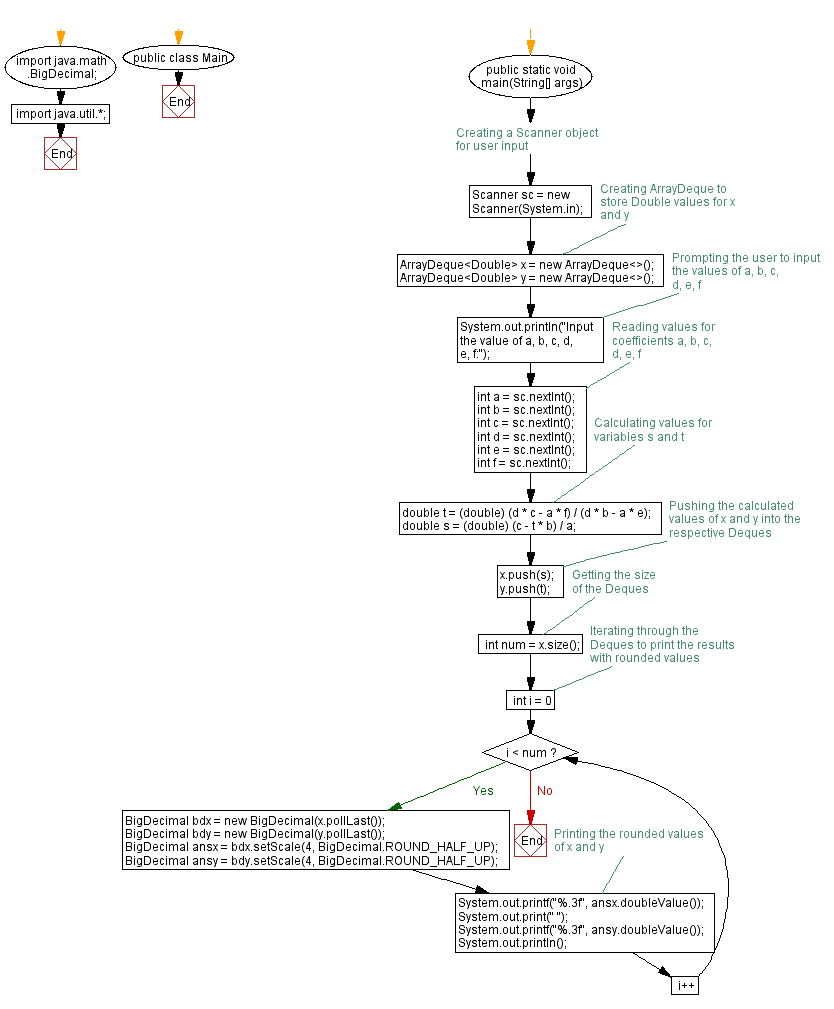# Java Exercises: Print the values of x, y where a, b, c, d, e and f are specified

## Java Basic: Exercise-214 with Solution

Write a Java program which solve the equation:
ax+by=c
dx+ey=f
Print the values of x, y where a, b, c, d, e and f are given.

Input:

a,b,c,d,e,f separated by a single space.
(-1,000 ≤ a,b,c,d,e,f ≤ 1,000)

Sample Solution:

Java Code:

``````import java.math.BigDecimal;
import java.util.*;
public class Main{
public static void main(String[] args) {
Scanner sc = new Scanner(System.in);
ArrayDeque<Double> x = new ArrayDeque<Double>();
ArrayDeque<Double> y = new ArrayDeque<Double>();
System.out.println("Input the value of a, b, c, d, e, f:");
int a = sc.nextInt();
int b = sc.nextInt();
int c = sc.nextInt();
int d = sc.nextInt();
int e = sc.nextInt();
int f = sc.nextInt();
double t = (double)(d*c-a*f)/(d*b-a*e);
double s = (double)(c-t*b)/a;
x.push(s);
y.push(t);
int num = x.size();
for(int i=0;i<num;i++){
BigDecimal bdx = new BigDecimal(x.pollLast());
BigDecimal bdy = new BigDecimal(y.pollLast());
BigDecimal ansx = bdx.setScale(4, BigDecimal.ROUND_HALF_UP);
BigDecimal ansy = bdy.setScale(4, BigDecimal.ROUND_HALF_UP);
System.out.printf("%.3f", ansx.doubleValue());
System.out.print(" ");
System.out.printf("%.3f", ansy.doubleValue());
System.out.println();
}
}
}
```
```

Sample Output:

```Input the value of a, b, c, d, e, f:
5 6 8 9 7 4
-1.684 2.737
```

Flowchart:Java Code Editor:

What is the difficulty level of this exercise?

﻿

## Java: Tips of the Day

Parsing dates:

```import java.io.*;
import java.util.*;
import java.text.*;

String s = "2001/09/23 14:39";

SimpleDateFormat formatter = new SimpleDateFormat ("yyyy/MM/dd H:mm");
Date d = formatter.parse(s, new ParsePosition(0));
```